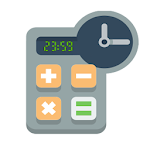• Author:

• Version:

Varies with device

• Update on:

## The description of Hours Minutes Calculator Time

Hours and Minutes Calculator, ( Hours Calculator )

With this time clev calculator you can perform the addition and subtraction operations between hours and minutes, it also allows you to multiply and divide those hours and minutes with integer numbers, for example:

1 hour and 36 minutes x 3 = 4 hours 48 minutes
2 hours and 15 minutes + 30 minutes * 2 = 5 hours 30 minutes
33 minutes / 2 + 1 hour and 56 minutes = 2 hours 12 minutes

Supports operations up to 99.999 hrs

You have 6 memory banks to save the result of hours and minutes of an operation, so you can use it later.

We have also added a history of operations so you never get lost and you can check the latest operations

I show case examples where this simple hour calculator is very practical:

* Time and attendance: How much working time? enter the time of departure and subtract the time of entry.
* Parking time control: Calculates the number of hours a vehicle has been in the parking lot.
* Hours tracker / hour tracker: to calculate hour minute of a task, your freetime, in time, to calculate if you did hour of extra.
* Calculate the duration of an event (duration calculator or hourstracker)
* To convert from standard time to military time, simply add 12 to the hour. So, if it's 5:30 p.m. standard time, you can add 12 to get the resulting 1730 in military time. To convert from military time to standard time, just subtract 12 from the hour, calculate hours.
* Time on any task, minutes in that period.
* Calculate the time until a specific point in time.

or simply use it as an hour calculator as time app.

This tool is widely used by people who work in places where they have to make calculations of hours, and with a simple and standard calculator it is not useful. As for example in human resources departments, where they often need to calculate the working hours / work hours of an employee, sometimes with several entries and exits in the same day, for example if an employee starts work at 08:15 and ends his working day at 17:08, it is not so easy to make these calculations, to know the worktime, if you do not have this tool used as a clevcalc app, aka clever calculator.

a time, a minute, an hour is not a problem, this time app CalcTime is specially adapted to combine them in mathematical time operations.